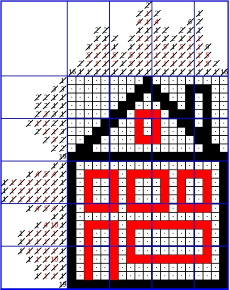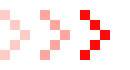INTRODUCTION
This example assumes that you are familiar with the basic steps that can be used to solve a nonogram as they are explained here. This example describes in more detail some steps that may be useful when the nonogram contains more than one colour. However, there are more logical steps you will need to use if you want to solve all nonograms!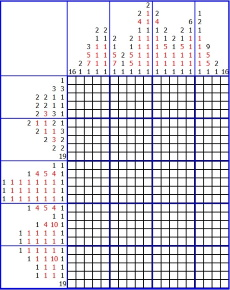BASIC PRINCIPLES
A nonogram consists of rows (horizontal) and columns (vertical), whereby in each row or column numbers indicate how many squares of a certain colour appear in that row or column. When a reference is made to a column number, then you need to start counting from the left most column (which is column number 1). When a reference is made to a row number, then you need to start counting from the top most row (which is row number 1).
For example: in column 2 you will find two black numbers (2 and 1). This means that somewhere in that column there is a range of 2 consecutive black squares and below it, separated by at least one white square, you will find a 'range' of one black square.
In column 3 there are two black numbers (3 and 1) and two red numbers (5 and 7). The order in which the numbers are given implies that, from top to bottom, there is a range of 3 black squares followed by a range of 5 red squares. Then there is range of 7 red squares and finally a 'range' of 1 black square.
You have to realise that it is not necessary to have a white square between ranges of different colours. They may be adjacent ranges. A white square is required between two ranges of the same colour.
In the program and in the example a dot means that it is known for sure that the square remains white.
HOW TO SOLVE A NONOGRAM WITH 2 COLOURS STEP 1
Consider the rows 10 and 25. In both rows there is one range with 19 black squares. Since the width of the nonogram is exactly 19 squares, this means that all squares in these rows are black. To indicate that we have completed a range of squares we put a diagonal line through the corresponding number.
When we consider the last row, then we see that in each of the columns 2 to 18 the last range is black and has length 1. We have found these ranges already and we can cross out the corresponding numbers. However, only the ranges in columns 2, 7 and 18 have another black range above them and therefore the square above them has to be white. So we can put a dot there. In the columns 3 to 6 and 8 to 18 we see that there is a red range above the black square, so the square above the black one may be red or it may be white. We don't know yet. The nonogram looks as follows: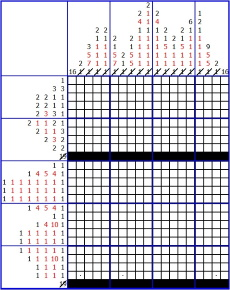HOW TO SOLVE A NONOGRAM WITH 2 COLOURS STEP 2
We now use the fact that the nonogram contains two colours. In column 3 we notice that there is a range of 7 consecutive red squares. When we look at the distribution of red squares over the rows, then we see that this range of 7 consecutive red squares can only be found between the rows 18 to 24. In all other cases the red range will cross a row which has only black squares.
The same applies to the red range with length 7 in column 6. So, we can colour these squares and cross out the corresponding numbers.
Notice that the red squares in rows 19 to 24 represent the first two red ranges of length 1. So, we can cross out their corresponding numbers, too, and place dots between the squares.
The result is as follows: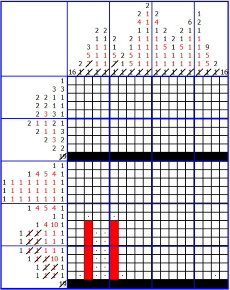HOW TO SOLVE A NONOGRAM WITH 2 COLOURS STEP 3
The same reasoning can be used for the red ranges with length 10 in the rows 18 and 22. They only fit between the columns 8 to 17.
This also applies to the red ranges with length 5 in columns 3 and 6. They only fit in between the rows 12 to 16.
In rows 13 - 15 the red squares represent the first two red ranges in these rows and therefore we can put dots in between them and cross out the corresponding numbers.
The next figure shows the result: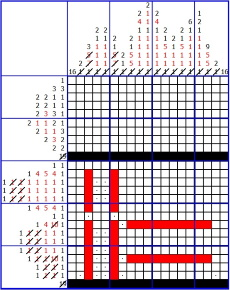HOW TO SOLVE A NONOGRAM WITH 2 COLOURS STEP 4
The red range with length 4 in row 18 only fits between the coloumns 3 to 6.
The same applies to the first red ranges with length 4 in rows 12 and 16.
In columns 8 and 17 we find two red ranges with length 5. In both cases the two ranges only fit between the rows 12 to 16 and the rows 18 to 22. We can cross out these numbers and put dots at various squares, which leads to the following result: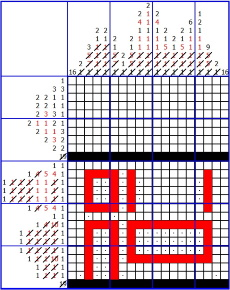HOW TO SOLVE A NONOGRAM WITH 2 COLOURS STEP 5
We continue to make use of the fact that the possibilities for placing red ranges are limited. In rows 12 and 16 the red ranges with length 5 and 4 only fit between the rows 8 to 12 and 14 to 17.
The red ranges with length 5 in columns 12 and 14 only fit between the rows 12 to 16.
Lastly, the black ranges with length 16 in columns 1 and 19 will only fit between the rows 10 to 25.
We cross out the appropriate numbers and put dots in the relevant squares and we have completed the bottom part of the nonogram.
This leads to the following result: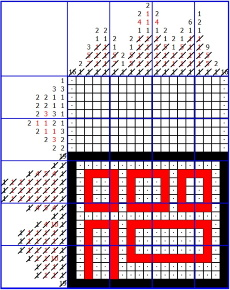HOW TO SOLVE A NONOGRAM WITH 2 COLOURS STEP 6
There are a few red ranges left and using the same line of thought as above we get the following result: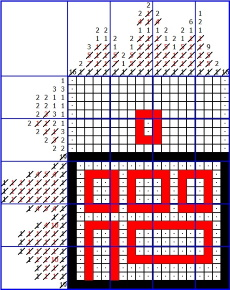FINAL RESULT
We can now complete the nonogram in the same way as is described in Example 1 and we get the following final result: